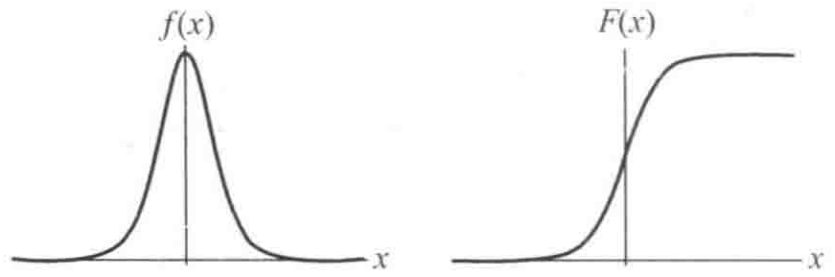# 逻辑斯谛回归

## 2. 逻辑斯谛分布

• 定义：设 $X$ 是连续随机变量，$X$ 服从逻辑斯谛分布是指 $X$ 具有下列分布函数和密度函数：

$F(x) = P(X \leq x) = \frac{1}{1 + e^{-(x-\mu)/\gamma}} \\ f(x) = F^{'}(x) = \frac{e^{-(x-\mu)/\gamma}}{\gamma(1 + e^{-(x-\mu)/\gamma})^2}$

其中，$\mu$ 是位置参数，$\gamma > 0$ 为形状参数。## 3. 二项逻辑斯谛回归模型

• 定义：二项逻辑斯谛回归模型是如下的条件概率分布：

$P(Y = 1 | x) = \frac{\exp(w \cdot x + b)}{1 + \exp(w \cdot x + b)} \\ P(Y = 0 | x) = \frac{1}{1 + \exp(w \cdot x + b)}$

其中，$x \in \mathbf{R}^n$ 为输入，$Y \in \{0, 1\}$ 为输出，$w \in \mathbf{R}^n$$b \in \mathbf{R}$ 为模型参数，$w$ 为权值向量，$b$ 为偏置，$w \cdot b$ 表示 $w$$b$ 的内积。

$\mathrm{logit}(p) = \log{\frac{p}{1-p}}$

$\log{\frac{P(Y = 1 | x)}{1 - P(Y = 1 | x)}} = w \cdot x + b$

• 参数估计：对于给定的训练数据集 $T = \{(x_1, y_1), (x_2, y_2), \cdots, (x_N, y_N)\}$，其中 $x_i \in \mathbf{R}^n$$y_i \in \{0, 1\}$，可以应用极大似然估计法估计二项逻辑斯谛模型参数。设 $P(Y = 1 | x) = \pi(x), P(Y = 0 | x) = 1 - \pi(x)$，似然函数为 $\prod_{i=1}^N [\pi(x_i)]^{y_i} [1-\pi(x_i)]^{1-y_i}$，则对数似然函数为

\begin{aligned} L(w) & = \sum_{i=1}^N [y_i \log{\pi(x_i)} + (1-y_i) \log{(1-\pi(x_i))}] \\ & = \sum_{i=1}^N \left[ y_i \log{\frac{\pi(x_i)}{1 - \pi(x_i)} + \log{(1-\pi(x_i))}} \right] \\ & = \sum_{i=1}^N [y_i (w \cdot x + b) - \log{(1 + \exp(w \cdot x +b))}] \end{aligned}

$L(w)$ 求极大值，即可得到 $w$ 的估计值。

$-L(w)$ 即对应交叉熵损失。

## 4. 多项逻辑斯谛回归

$P(Y = k | x) = \frac{\exp(w_k \cdot x)}{1 + \sum_{k=1}^{K-1} \exp(w_k \cdot x)}, k = 1, 2, \cdots, K-1 \\ P(Y = K | x) = \frac{1}{1 + \sum_{k=1}^{K-1} \exp(w_k \cdot x)}$

## 附录

• 《统计学习方法》by 李航